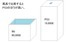# Unresolved Detail In Plotted Equations

Sometimes the calculator detects that an equation is too complicated to plot perfectly in a reasonable amount of time. When this happens, the equation is plotted at lower resolution.

Was this article helpful?
221 out of 246 found this helpful
Have more questions? Submit a request

#### 927 Comments

•Kelvingtonib

x^2y^2=\left(\tan \left(\pi ^{y^{\frac{2}{5}}}\right)\right)

This creates two perfect gradients

•Jake browning

Or: e^(log(x)*y)=999

•Colby Phillips

sin x =sin y

is super cool!

•Kbasinger21

\frac{5x^y}{3y^x}=\sin \left(\cos \left(x\right)\right)

•Jason Husvar

y^sinx=x^siny

put in sin(cos(tan(x)))=sin(cos(tan(y))) and sin(cos(tan(csc(sec(cot(x))))))=sin(cos(tan(csc(sec(cot(y)))))) in two different equations at one time.

I then broke my computer doing the same as the second part of the above comment, but put each of the trig functions in twice

•Kbasinger21

xy^{\sin xy}=xy^{\cos xy} then zoom out

•MattTheWaffleCat

It gets crazy when you .05\ge \frac{\left(\frac{\frac{\tan x^2}{\sin x^2}}{\cos \ x^2}+\frac{\frac{\tan y^2}{\sin x^2}}{\cos x^2}\right)}{\left(\tan x^3\right)\cdot \left(\tan y^3\right)}

•Gavin R

100=\frac{\frac{\frac{\left(\frac{\left(\left(\sin \left(x+3\right)\left(\cos x-8\right)\left(\tan \left(x-8\right)^2\right)\right)\right)}{\left(x-7\right)\left(x-4\right)\left(x+2\right)\left(x-y\right)}\right)\left(y^{\left(\left(2\right)x\right)}\right)}{\left(x-\tan \left(x+y\right)\right)\left(y-\tan \left(x+y\right)\right)}\left(ye\pi \right)}{y^x+x^y+\pi }\left(\frac{\left(\frac{\left(y\left(\log _xy\right)^{\sin \left(x\right)}\right)}{y+6-\frac{4\sin \left(x\right)}{\log _xy}}\right)\left(\frac{\left(\frac{e}{\pi }+\frac{x}{e}\right)}{\left(\tan \left(e\right)+\sin \left(e\right)+\cos \left(e\right)\right)\pi }\right)}{\log _91000y+ye\pi }\right)ye\pi \left(\sin \left(\frac{x}{y}\right)\right)}{\sin \left(xyxyxyx\right)\log _xy}\left(\frac{\frac{\frac{\frac{\left(\frac{\cos \left(7x+3\right)}{\tan \left(6x\right)}\left(\sin \left(x^3\right)\right)-\frac{x}{\cos \left(7x^5\right)}\right)}{x^3\left(\frac{\cos \left(x+2\right)}{\sin \left(\frac{x}{7}\right)}\right)}}{y\left(\tan \left(\frac{x}{x-6}\right)+\cos \left(x\right)\right)}\left(\log _xy\right)\left(\frac{\tan \left(xy\right)\sin \left(\frac{x}{y}\right)\cos \left(\frac{y}{x}\right)}{\frac{\tan \left(\frac{y}{x}\right)\sin \left(xy\right)\cos \left(\frac{x}{y}\right)}{\tan \left(\frac{x}{y}\right)\sin \left(\frac{y}{x}\right)\cos \left(xy\right)}}\left(e^x\right)\right)}{\left(\tan ex\right)\left(\log _{7x}y\right)\left(god\right)}\left(\log _{\tan x}y\right)}{e}\left(e^6+y^2+x^2+g^2+o^2+d^2\right)^3\right)

I have no idea what I'm doing.

•Ethan Gilchrist
•Dante Demerath

\sin \left(x!\right)=\cos \left(y^{y!}\right)

opens a portal to another dimention

•伊藤那由多

Even sin(2pix)sin(2piy)=0 showed the message... It's quite obvious that this is a grid.

•Henry Lee
•cameron broberg

I found y=x/z graphed on the same axis as z=x/y to be very interesting. It can be simplified to say 1=1, so it should graph every point, but it instead forms a pattern of unresolved detail. Likely a result of the program and not the math.

•Mcl0011

Never try sin(xy)-cos(xy)-tan(xy)=sin(xy)+cos(xy)+tan(xy)

•104989

this is COMPLETELY insane... also you can use this to make a sunflower :)

\tan \left(999^{x^{\frac{1}{3}}y^{\frac{1}{3}}}\right)=\sin \left(999^9x^2y\right)\left\{.01\le x^2+y^2\le 4\right\}

•Az

cos(x)<1, oddly, when you zoom out

•Az

sin(x)=x^(xx) shows nothing but pops the message up

•Az

\sin \left(\cos \left(\tan x\right)\right)=\sin \left(\cos \left(\tan y\right)\right) looks beautiful

•Pinrui Huang

0\le \sin \left(-3x^2+2y\right)

•Fabian Rehhaus

y=\sqrt[\left|x!\right|]{y!}\cdot \sin \left(y\right)

•20avva

or just x!=y!

•Lone Stars

yet some how \csc \left(\tan \left(\tan \left(\tan \left(\tan \left(5x+1\right)\right)\right)\right)\right) isnt to detailed to have dis happen.... also RUNWAY BABY XD

•20avva

x\sin x^y=1

•Usbstickuser

x=y cos(xy) takes it to a whole new level in a beautiful way though

•Oon Han

\tan \left(\frac{x}{y}\right)=\cos \left(\frac{x}{y}\right)\sin \left(\frac{x}{y}\right)

•Computingpotatochips

y=\frac{\left(4\left(0.5x\right)+\frac{2^x}{x^2-\frac{3x^4}{x^x}}\cdot \cos \left(\frac{\pi x}{3}\right)^x\right)}{\ln \left(\frac{2x}{\sin \left(7x^4\right)-\cos \left(\pi ^{\frac{x}{2x}}\right)}\right)}+\frac{\tan \left(\frac{x^{\pi }}{3}\right)}{2x^4-\frac{3x}{\log \left(2\right)}}+3

•Computingpotatochips

Try altering c to change adjustable fine detail. https://www.desmos.com/calculator/hhaqt3tpxr

•Neeleyl9565

Lag Machine 9000: xx\sin \left(\tan \left(\sqrt{xyxxyx}\right)\right)y\ =\ yy\sin \left(\tan \left(\sqrt{xyxxyx}\right)\right)x

crescent thing: x!y\sin \left(y^x\right)x^{yyy}=y!x\sin \left(x^y\right)y^{xxx}

Lag Machine 9001: \left|xyyx\right|=\left|yxxy\right|

Trailing Off Functions (doesnt lag or give unresolved details, the function just looks neat): x^{x^{x^{x^{x^{x^{x^{x^{x^x}}}}}}}}\sin \left(x^{x^{x^{x^{x^{x^{x^{x^{x^x}}}}}}}}\right)=y^{y^{y^{y^{y^{y^{y^{y^{y^y}}}}}}}}\sin \left(y^{y^{y^{y^{y^{y^{y^{y^{y^y}}}}}}}}\right)

•Neeleyl9565

Supernova: \sin \left(\cos \left(\tan \left(\frac{\frac{x}{2}x}{\frac{y}{2}y}+\cos \left(\frac{3}{10}y\right)\right)\right)\right)\ =\ yx

A Hole In The Universe: \tan \left(\frac{\sin \left(x\right)y}{\cos \left(y\right)x}\right)!!<xyxy

•Urav Maniar
Article is closed for comments.